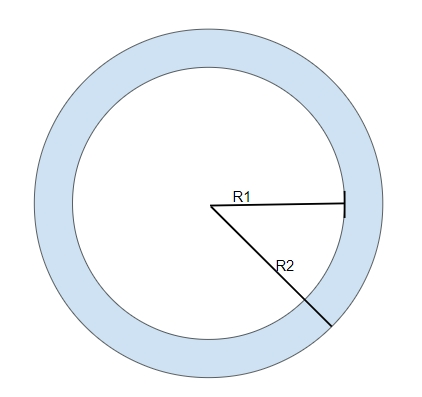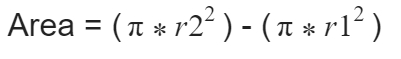# Program to calculate the area between two Concentric Circles in C++?

C++Server Side ProgrammingProgramming

#### C in Depth: The Complete C Programming Guide for Beginners

45 Lectures 4.5 hours

#### Practical C++: Learn C++ Basics Step by Step

Most Popular

50 Lectures 4.5 hours

#### Master C and Embedded C Programming- Learn as you go

66 Lectures 5.5 hours

## What is Concentric Circle?

Concentric circle is the circle inside the circle which means they share common center with different radius lengths i.e. r1 and r2 where, r2>r1. Region between two concentric circles is known as annulus.

Given below is the figure of Concentric Circle## Problem

Given with two concentric circles of different radius lengths r1 and r2 where r2>r1. The task is to find the area between both the circles which is highlighted by the blue colour.

To calculate area between two circles we can subtract the area of larger circle from smaller circle

Lets say, bigger circle have radius r2 and smaller circle have radius of length r1 than## Example

Input-: r1=3 r2=4
Output-: area between two given concentric circle is :21.98

## Algorithm

Start
Step 1 -> define macro as
#define pi 3.14
Step 2 -> Declare function to find area between the two given concentric circles
double calculateArea(int x, int y)
set double outer = pi * x * x
Set double inner = pi * y * y
return outer-inner
step 3 -> In main()
Declare variable as int x = 4 and int y = 3
Print calculateArea(x,y)
Stop

## Example

#include <bits/stdc++.h>
#define pi 3.14
using namespace std;
// Function to find area between the two given concentric circles
double calculateArea(int x, int y){
double outer = pi * x * x;
double inner = pi * y * y;
return outer-inner;
}
int main(){
int x = 4;
int y = 3;
cout <<"area between two given concentric circle is :"<<calculateArea(x, y);
return 0;
}

## Output

area between two given concentric circle is :21.98